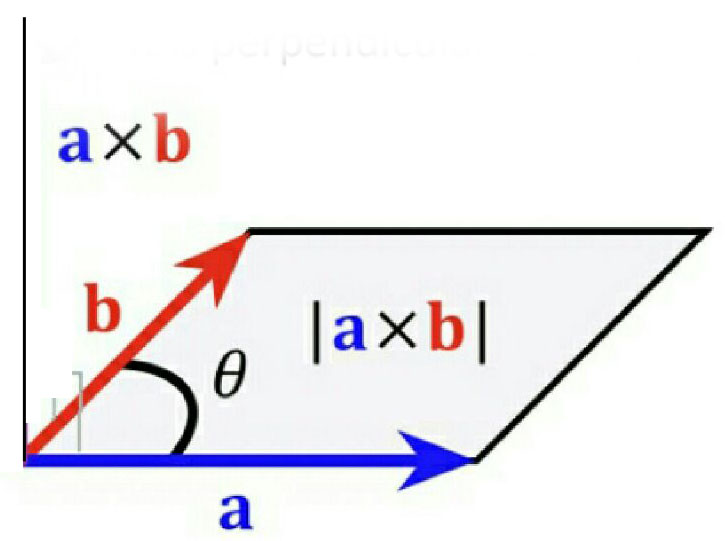#### Ifthen which of the following statement is not true? Option 1)Option 2)Option 3)Option 4)As we discussed in

Vector or cross product -

Vector or cross product of two vector&written asis a single vector whose magnitude is equal to product of magnitude of&and the sine of smaller anglebetween them.- whereinFigure 6 shows representation of vector or cross product of vectors.

shows representation of vector or cross product of vectors

Form the property of vector product, we notice thatmust be perpendicular to the plane formed by vectorand. Thusis Perpendicular to bothandandVector also.

Option 1)Option is Incorrect

Option 2)Option is Incorrect

Option 3)Option is Incorrect

Option 4)Option is Correct